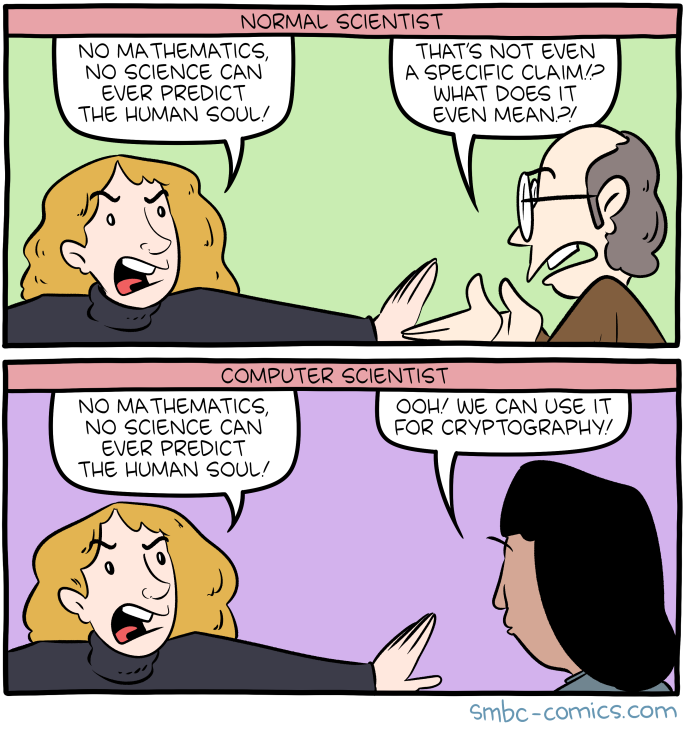## Aside: Knapsack Crypto-system

Given that we used LLL to tackle a knapsack-style problem yesterday. You might every now and again hear about the Knapsack Cryptosystem. It died when LLL came out. BUT it is proof of the SMBC comic:I have seen it in 1 CTF in the last year, and crushed it with LLL.

Likewise I saw 2 CTF problems which needed to be solved with Shortest Vector Problem / Closest Vector Problem. Again solved with LLL.

# Class 20: Coppersmith's Method/Attack

This series of attacks is very clever and uses LLL in a very general fashion.

The big idea is "Coppersmith's Method" which uses LLL to find solutions to $$f(x) = 0$$ mod $$N$$ for small values of $$x$$.

1. 1) Learn HOW Coppersmith's method finds small roots of polynomials mod something.
2. 2) Learn HOW YOU CAN USE Coppersmith's method (now Attack) to break RSA-based problems

Given that this is Applied Cryptography I'm going to peak through door #1 then actually go with door #2.

# DOOR 1 TLDR: How it works, Why it matters:

1) "Any integer which is small enough has to be zero."

2) Finding roots of polynomials over the integers is EASY.

3) Finding roots mod N is HARD.

4) Finding roots mod N is useful. Most useful things can be expressed as solutions to polynomials mod N. For instance: if $$m^e = C$$ mod N. Then you crack RSA if you have a solution to $$x^e - C = 0$$ mod N.

4.1) If you think you know part of the message $$m$$, like you know the padding, suppose $$m$$ has the format "The flag is ninja{UNKNOWN_STUFF_HERE}". Then you can try to "solve" $$(p+x)^e - C = 0$$ where $$p$$ (the pad) is that stuff you know.

4.2) If you think you know part of $$q$$ a factor of $$N$$ then you could "solve" $$x-\bar{q}$$ mod N. Where $$\bar{q}$$ is your guess at $$q$$.

5) HOW it works. Make a family of polynomials that all share the roots of your polynomial mod $$N^a$$ for some $$a$$.

5.1) Use LLL to find a combination of those polynomials with "SMALL" coefficients.

5.2) If that polynomial you create, which has all of the roots of $$f$$ but is now mod $$N^a$$ and has "small" coefficients, then find its roots over the INTEGERS and check them for your original problem.

5.3) Tweak parameters until you win or decide no small roots exist.

Best Coppersmith paper in the world, IMHO: Alexander May's PhD Thesis (what a beautiful PhD).

# Door 2: Actually Doing It

Here is the problem we will solve:

Now I want to spend the rest of our time using a decent implementation to solve a real problem: